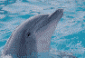# curious lack of warning/error

5 posts / 0 newOffline
Joined: 10/19/2009 - 15:38
curious lack of warning/error
AttachmentSizecuriousProblem.R1.99 KBdz.csv1.58 KB

In fitting a bivariate threshold model using a definition variable, OpenMx converged but the final result gave the following predicted covariance matrix
InitT1 InitT2
InitT1 1.00000 1.48088
InitT2 1.48088 1.00000

Obviously not positive definite. Yet I did not get any warnings or errors.

Script and data attached.

GregOffline
Joined: 10/19/2009 - 15:38
PS Could the problem be in

PS Could the problem be in omxMnor? Add the following line to the code in my previous post:
omxMnor(preCov, c(0, 0), c(-Inf, -Inf), c(t, t))Offline
Joined: 07/31/2009 - 15:14
It's a bug!

I believe this is a bug. omxMnor() seems to know what to do with correlation matrices only. I don't know what it is doing otherwise. Nor am I sure what it is doing with the non-pd input.

> omxMnor(matrix(c(1,0,0,1),2,2), c(0, 0), c(-Inf, -Inf), c(0,0))
[,1]
[1,] 0.25
> omxMnor(matrix(c(1,0,0,1),2,2), c(0, 0), c(-Inf, -Inf), c(1.282,1.282))
[,1]
[1,] 0.8101416
> omxMnor(matrix(c(1,90,90,1),2,2), c(0, 0), c(-Inf, -Inf), c(1.282,1.282))
[,1]
[1,] 0.5056822
> omxMnor(matrix(c(1,90,1,90),2,2), c(0, 0), c(-Inf, -Inf), c(1.282,1.282))
[,1]
[1,] 0.5370363Offline
Joined: 07/31/2009 - 15:14
Well maybe it's a bug

The following do check out ok against classic Mx. I suspect that a) OpenMx only pays attention to the triangle below the diagonal of the covariance matrix (second example); and b) that it does something to curtail out-of-range values for the correlation so that optimization doesn't come to a grinding halt. I've logged a bug report: http://openmx.psyc.virginia.edu/issue/2013/02/omxmnor-problem
and also fixed a couple of minor errors in the example script (reading & mxFactoring the data)

> omxMnor(matrix(c(1,.5,.5,1),2,2), c(0, 0), c(-Inf, -Inf), c(0,0))
[,1]
[1,] 0.3333333
> omxMnor(matrix(c(1,.3,.5,1),2,2), c(0, 0), c(-Inf, -Inf), c(1, 1))
[,1]
[1,] 0.7281473
> omxMnor(matrix(c(1,.3,.3,1),2,2), c(0, 0), c(-Inf, -Inf), c(1, 1))
[,1]
[1,] 0.7281473

I don't see anything wrong with the standardization code (in omxAlgebraFunctions.c) called before the call to SADMVN to get the integral:

void omxStandardizeCovMatrix(omxMatrix* cov, double* corList, double* weights) {
// Maybe coerce this into an algebra or sequence of algebras?

if(OMX_DEBUG) { Rprintf("Standardizing matrix."); }

int rows = cov->rows;

for(int i = 0; i < rows; i++) {
weights[i] = sqrt(omxMatrixElement(cov, i, i));
}

for(int i = 0; i < rows; i++) {
for(int j = 0; j < i; j++) {
corList[((i*(i-1))/2) + j] = omxMatrixElement(cov, i, j) / (weights[i] * weights[j]);
}
}
}Offline
Joined: 10/19/2009 - 15:38
Any fix soon? Bet many users

Any fix soon? Bet many users struggling with IFAIL=6 messages for threshold models will appreciate it while (shudder) some with models that converged may have to redo their (shudder even more, published) results.

In the interim, suggest using constraints to make certain the matrix is pd.